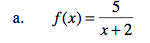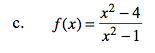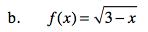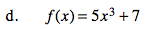### Home > PC > Chapter 2 > Lesson 2.3.2 > Problem2-89

2-89.
1. Find the domain of each function. Homework Help ✎

1. f(x) =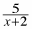2. f(x) =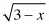3. f(x) =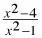4. f(x) = 5x3 + 7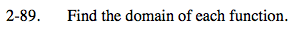The domain is all of the possible x-values for which an output of the function exists. Consider values where x causes division by zero or a negative number inside an even root.